Next: About this document ... Up: APPENDIX: POTENTIAL, FIELD AND Previous: Field Components

## Radiated Momentum

Being generated by a circular loop, the density of radiated momentum pointing into the
direction is independent of the polar angle
. Consequently, that density's spatial integral, Eq.(66), reduces to
(86)

The
-integration affects only the dotted factors, and they vanish outside a sufficiently large-interval, i.e.
. Consequently, integration by parts yields
andThus there remain only three non-zero terms in the integral,(87)

The coefficients of the squared terms are
(88)(89)(90)

We now take advantage of the fact that the integral, Eq.(87), is independent of the synchronous time
. This simplifies the evaluation of the integral considerably because one may assume
without changing the value of the integral. The final outcome is that (i) in each of the expressions, Eqs.(88)-(90), only the last term contributes to the-integral and (ii) the integral assumes a simple mathematical form if one introduces
as the new integration variable. With this scheme one has
(91)

The to-be-used integrands have the form
both of which are always less than one in absolute value, even when they get multiplied by
. Consequently, one is perfectly justified in saying that
(92)

Taking note that only the last terms of Eqs.(88)-(90) give nonzero contribution, apply the limiting form, Eq.(92), to evaluate the integral, Eq.(87). One finds that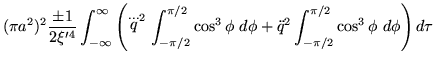(93)

The value of the integral
implies that the final result is
(94)

the total momentum into the-direction radiated by a magnetic dipole accelerated in Rindler sector
(upper sign) or in Rindler sector(lower sign). This is the result stated by Eq.(66)Next: About this document ... Up: APPENDIX: POTENTIAL, FIELD AND Previous: Field Components
Ulrich Gerlach 2001-10-09